# 三、分类

## MNIST

Scikit-Learn 提供了许多辅助函数，以便于下载流行的数据集。MNIST 是其中一个。下面的代码获取 MNIST

>>> from sklearn.datasets import fetch_mldata
>>> mnist = fetch_mldata('MNIST original')
>>> mnist
{'COL_NAMES': ['label', 'data'],
'DESCR': 'mldata.org dataset: mnist-original',
'data': array([[0, 0, 0, ..., 0, 0, 0],
[0, 0, 0, ..., 0, 0, 0],
[0, 0, 0, ..., 0, 0, 0],
...,
[0, 0, 0, ..., 0, 0, 0],
[0, 0, 0, ..., 0, 0, 0],
[0, 0, 0, ..., 0, 0, 0]], dtype=uint8),
'target': array([ 0., 0., 0., ..., 9., 9., 9.])}


>>> X, y = mnist["data"], mnist["target"]
>>> X.shape
(70000, 784)
>>> y.shape
(70000,)


MNIST 有 70000 张图片，每张图片有 784 个特征。这是因为每个图片都是28*28像素的，并且每个像素的值介于 0~255 之间。让我们看一看数据集的某一个数字。你只需要将某个实例的特征向量，reshape28*28的数组，然后使用 Matplotlib 的imshow函数展示出来。


%matplotlib inline
import matplotlib
import matplotlib.pyplot as plt
some_digit = X
some_digit_image = some_digit.reshape(28, 28)
plt.imshow(some_digit_image, cmap = matplotlib.cm.binary, interpolation="nearest")
plt.axis("off")
plt.show()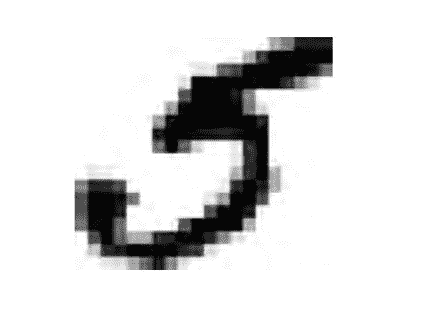>>> y
5.0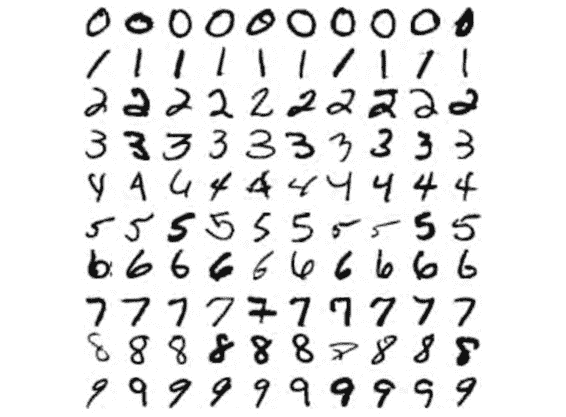X_train, X_test, y_train, y_test = X[:60000], X[60000:], y[:60000], y[60000:]


import numpy as np

shuffle_index = np.random.permutation(60000)
X_train, y_train = X_train[shuffle_index], y_train[shuffle_index]


## 训练一个二分类器

y_train_5 = (y_train == 5) # True for all 5s, False for all other digits.
y_test_5 = (y_test == 5)


from sklearn.linear_model import SGDClassifier
sgd_clf = SGDClassifier(random_state=42)
sgd_clf.fit(X_train, y_train_5)


SGDClassifier依赖于训练集的随机程度（所以被命名为 stochastic，随机之义）。如果你想重现结果，你应该固定参数random_state

>>> sgd_clf.predict([some_digit])
array([ True], dtype=bool)


## 对性能的评估

### 实现交叉验证

from sklearn.model_selection import StratifiedKFold
from sklearn.base import clone
skfolds = StratifiedKFold(n_splits=3, random_state=42)
for train_index, test_index in skfolds.split(X_train, y_train_5):
clone_clf = clone(sgd_clf)
X_train_folds = X_train[train_index]
y_train_folds = (y_train_5[train_index])
X_test_fold = X_train[test_index]
y_test_fold = (y_train_5[test_index])
clone_clf.fit(X_train_folds, y_train_folds)
y_pred = clone_clf.predict(X_test_fold)
n_correct = sum(y_pred == y_test_fold)
print(n_correct / len(y_pred)) # prints 0.9502, 0.96565 and 0.96495


StratifiedKFold类实现了分层采样（详见第二章的解释），生成的折（fold）包含了各类相应比例的样例。在每一次迭代，上述代码生成分类器的一个克隆版本，在训练折（training folds）的克隆版本上进行训练，在测试折（test folds）上进行预测。然后它计算出被正确预测的数目和输出正确预测的比例。

>>> from sklearn.model_selection import cross_val_score
>>> cross_val_score(sgd_clf, X_train, y_train_5, cv=3, scoring="accuracy")
array([ 0.9502 , 0.96565, 0.96495]


from sklearn.base import BaseEstimator
class Never5Classifier(BaseEstimator):
def fit(self, X, y=None):
pass
def predict(self, X):
return np.zeros((len(X), 1), dtype=bool)


>>> never_5_clf = Never5Classifier()
>>> cross_val_score(never_5_clf, X_train, y_train_5, cv=3, scoring="accuracy")
array([ 0.909 , 0.90715, 0.9128 ])


### 混淆矩阵

from sklearn.model_selection import cross_val_predict
y_train_pred = cross_val_predict(sgd_clf, X_train, y_train_5, cv=3)


>>> from sklearn.metrics import confusion_matrix
>>> confusion_matrix(y_train_5, y_train_pred)
array([[53272, 1307],
[ 1077, 4344]])


>>> confusion_matrix(y_train_5, y_train_perfect_predictions)
array([[54579, 0],
[ 0, 5421]])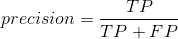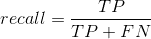FN是假反例的数目。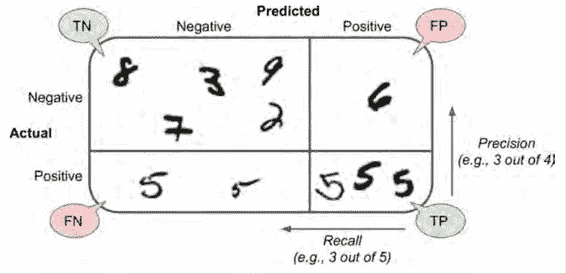### 准确率与召回率

Scikit-Learn 提供了一些函数去计算分类器的指标，包括准确率和召回率。

>>> from sklearn.metrics import precision_score, recall_score
>>> precision_score(y_train_5, y_pred) # == 4344 / (4344 + 1307)
0.76871350203503808
>>> recall_score(y_train_5, y_train_pred) # == 4344 / (4344 + 1077)
0.79136690647482011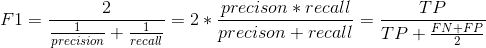>>> from sklearn.metrics import f1_score
>>> f1_score(y_train_5, y_train_pred)
0.78468208092485547


F1 支持那些有着相近准确率和召回率的分类器。这不会总是你想要的。有的场景你会绝大程度地关心准确率，而另外一些场景你会更关心召回率。举例子，如果你训练一个分类器去检测视频是否适合儿童观看，你会倾向选择那种即便拒绝了很多好视频、但保证所保留的视频都是好（高准确率）的分类器，而不是那种高召回率、但让坏视频混入的分类器（这种情况下你或许想增加人工去检测分类器选择出来的视频）。另一方面，加入你训练一个分类器去检测监控图像当中的窃贼，有着 30% 准确率、99% 召回率的分类器或许是合适的（当然，警卫会得到一些错误的报警，但是几乎所有的窃贼都会被抓到）。

### 准确率/召回率之间的折衷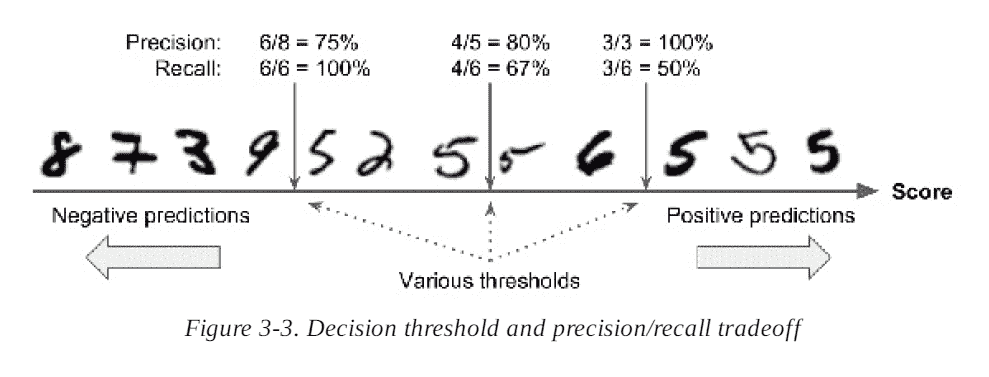Scikit-Learn 不让你直接设置阈值，但是它给你提供了设置决策分数的方法，这个决策分数可以用来产生预测。它不是调用分类器的predict()方法，而是调用decision_function()方法。这个方法返回每一个样例的分数值，然后基于这个分数值，使用你想要的任何阈值做出预测。

>>> y_scores = sgd_clf.decision_function([some_digit])
>>> y_scores
array([ 161855.74572176])
>>> threshold = 0
>>> y_some_digit_pred = (y_scores > threshold)
array([ True], dtype=bool)


SGDClassifier用了一个等于 0 的阈值，所以前面的代码返回了跟predict()方法一样的结果（都返回了true）。让我们提高这个阈值：

>>> threshold = 200000
>>> y_some_digit_pred = (y_scores > threshold)
>>> y_some_digit_pred
array([False], dtype=bool)


y_scores = cross_val_predict(sgd_clf, X_train, y_train_5, cv=3,
method="decision_function")


from sklearn.metrics import precision_recall_curve
precisions, recalls, thresholds = precision_recall_curve(y_train_5, y_scores)


def plot_precision_recall_vs_threshold(precisions, recalls, thresholds):
plt.plot(thresholds, precisions[:-1], "b--", label="Precision")
plt.plot(thresholds, recalls[:-1], "g-", label="Recall")
plt.xlabel("Threshold")
plt.legend(loc="upper left")
plt.ylim([0, 1])
plot_precision_recall_vs_threshold(precisions, recalls, thresholds)
plt.show()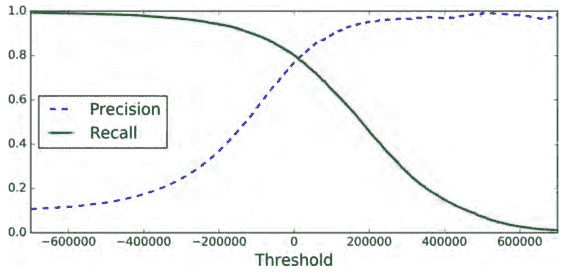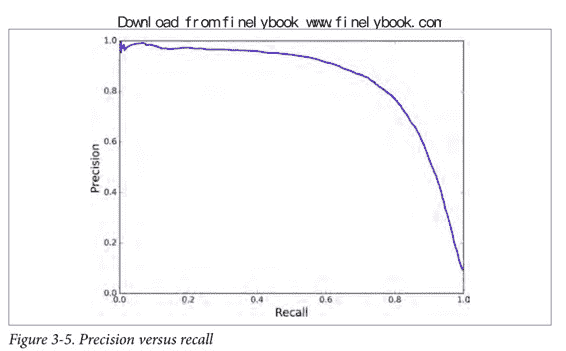y_train_pred_90 = (y_scores > 70000)


>>> precision_score(y_train_5, y_train_pred_90)
0.8998702983138781
>>> recall_score(y_train_5, y_train_pred_90)
0.63991883416343853


### ROC 曲线

from sklearn.metrics import roc_curve
fpr, tpr, thresholds = roc_curve(y_train_5, y_scores)


def plot_roc_curve(fpr, tpr, label=None):
plt.plot(fpr, tpr, linewidth=2, label=label)
plt.plot([0, 1], [0, 1], 'k--')
plt.axis([0, 1, 0, 1])
plt.xlabel('False Positive Rate')
plt.ylabel('True Positive Rate')
plot_roc_curve(fpr, tpr)
plt.show()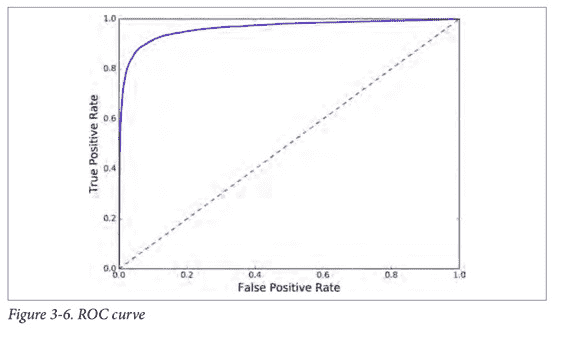>>> from sklearn.metrics import roc_auc_score
>>> roc_auc_score(y_train_5, y_scores)
0.97061072797174941


from sklearn.ensemble import RandomForestClassifier
forest_clf = RandomForestClassifier(random_state=42)
y_probas_forest = cross_val_predict(forest_clf, X_train, y_train_5, cv=3,
method="predict_proba")


y_scores_forest = y_probas_forest[:, 1] # score = proba of positive class
fpr_forest, tpr_forest, thresholds_forest = roc_curve(y_train_5,y_scores_forest)


plt.plot(fpr, tpr, "b:", label="SGD")
plot_roc_curve(fpr_forest, tpr_forest, "Random Forest")
plt.legend(loc="bottom right")
plt.show()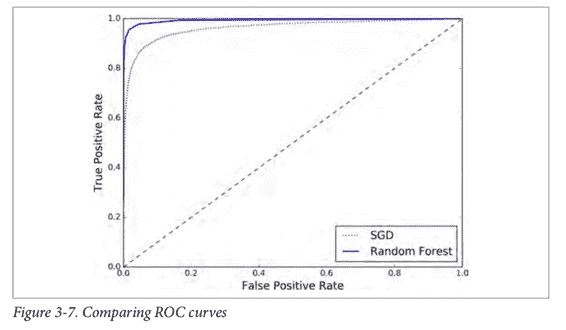>>> roc_auc_score(y_train_5, y_scores_forest)
0.99312433660038291


## 多类分类

Scikit-Learn 可以探测出你想使用一个二分类器去完成多分类的任务，它会自动地执行 OvA（除了 SVM 分类器，它使用 OvO）。让我们试一下SGDClassifier.

>>> sgd_clf.fit(X_train, y_train) # y_train, not y_train_5
>>> sgd_clf.predict([some_digit])
array([ 5.])


>>> some_digit_scores = sgd_clf.decision_function([some_digit])
>>> some_digit_scores
array([[-311402.62954431, -363517.28355739, -446449.5306454 ,
-183226.61023518, -414337.15339485, 161855.74572176,
-452576.39616343, -471957.14962573, -518542.33997148,
-536774.63961222]])


>>> np.argmax(some_digit_scores)
5
>>> sgd_clf.classes_
array([ 0., 1., 2., 3., 4., 5., 6., 7., 8., 9.])
>>> sgd_clf.classes_
5.0


>>> from sklearn.multiclass import OneVsOneClassifier
>>> ovo_clf = OneVsOneClassifier(SGDClassifier(random_state=42))
>>> ovo_clf.fit(X_train, y_train)
>>> ovo_clf.predict([some_digit])
array([ 5.])
>>> len(ovo_clf.estimators_)
45


>>> forest_clf.fit(X_train, y_train)
>>> forest_clf.predict([some_digit])
array([ 5.])


>>> forest_clf.predict_proba([some_digit])
array([[ 0.1, 0. , 0. , 0.1, 0. , 0.8, 0. , 0. , 0. , 0. ]])


>>> cross_val_score(sgd_clf, X_train, y_train, cv=3, scoring="accuracy")
array([ 0.84063187, 0.84899245, 0.86652998])


>>> from sklearn.preprocessing import StandardScaler
>>> scaler = StandardScaler()
>>> X_train_scaled = scaler.fit_transform(X_train.astype(np.float64))
>>> cross_val_score(sgd_clf, X_train_scaled, y_train, cv=3, scoring="accuracy")
array([ 0.91011798, 0.90874544, 0.906636 ])


## 误差分析

>>> y_train_pred = cross_val_predict(sgd_clf, X_train_scaled, y_train, cv=3)
>>> conf_mx = confusion_matrix(y_train, y_train_pred)
>>> conf_mx
array([[5725, 3, 24, 9, 10, 49, 50, 10, 39, 4],
[ 2, 6493, 43, 25, 7, 40, 5, 10, 109, 8],
[ 51, 41, 5321, 104, 89, 26, 87, 60, 166, 13],
[ 47, 46, 141, 5342, 1, 231, 40, 50, 141, 92],
[ 19, 29, 41, 10, 5366, 9, 56, 37, 86, 189],
[ 73, 45, 36, 193, 64, 4582, 111, 30, 193, 94],
[ 29, 34, 44, 2, 42, 85, 5627, 10, 45, 0],
[ 25, 24, 74, 32, 54, 12, 6, 5787, 15, 236],
[ 52, 161, 73, 156, 10, 163, 61, 25, 5027, 123],
[ 43, 35, 26, 92, 178, 28, 2, 223, 82, 5240]])


plt.matshow(conf_mx, cmap=plt.cm.gray)
plt.show()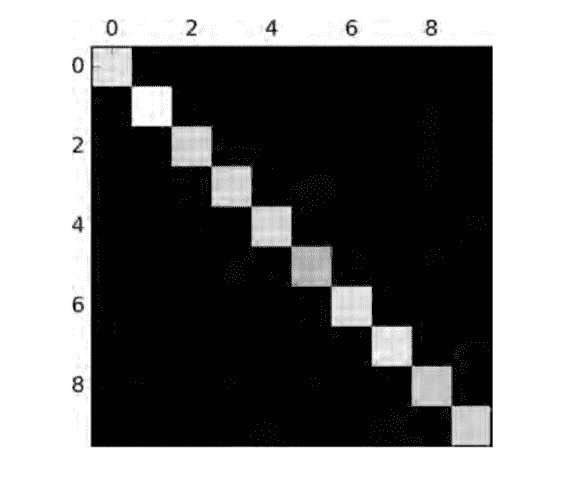row_sums = conf_mx.sum(axis=1, keepdims=True)
norm_conf_mx = conf_mx / row_sums


np.fill_diagonal(norm_conf_mx, 0)
plt.matshow(norm_conf_mx, cmap=plt.cm.gray)
plt.show()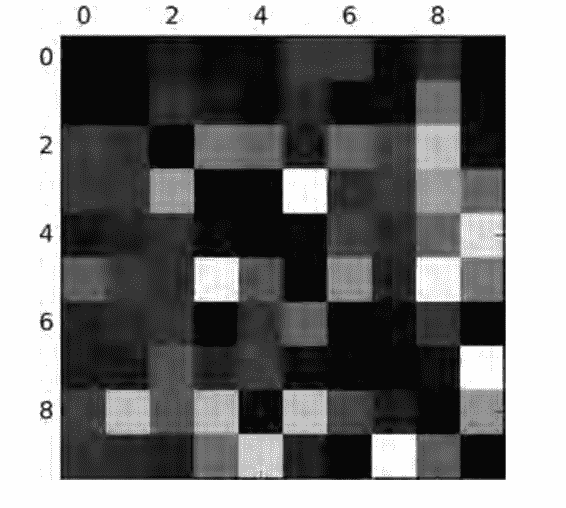cl_a, cl_b = 3, 5
X_aa = X_train[(y_train == cl_a) & (y_train_pred == cl_a)]
X_ab = X_train[(y_train == cl_a) & (y_train_pred == cl_b)]
X_ba = X_train[(y_train == cl_b) & (y_train_pred == cl_a)]
X_bb = X_train[(y_train == cl_b) & (y_train_pred == cl_b)]
plt.figure(figsize=(8,8))
plt.subplot(221); plot_digits(X_aa[:25], ../images_per_row=5)
plt.subplot(222); plot_digits(X_ab[:25], ../images_per_row=5)
plt.subplot(223); plot_digits(X_ba[:25], ../images_per_row=5)
plt.subplot(224); plot_digits(X_bb[:25], ../images_per_row=5)
plt.show()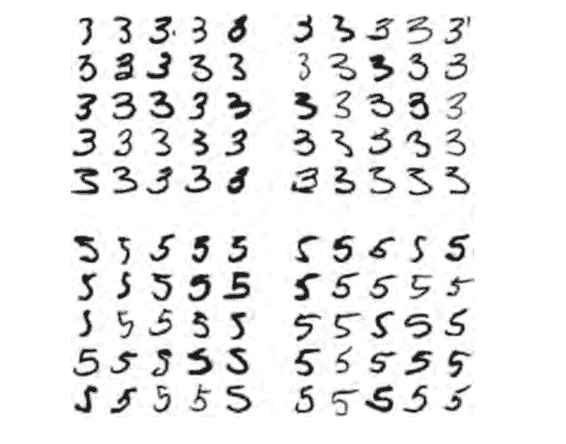3 和 5 之间的主要差异是连接顶部的线和底部的线的细线的位置。如果你画一个 3，连接处稍微向左偏移，分类器很可能将它分类成 5。反之亦然。换一个说法，这个分类器对于图片的位移和旋转相当敏感。所以，减轻3/5混淆的一个方法是对图片进行预处理，确保它们都很好地中心化和不过度旋转。这同样很可能帮助减轻其他类型的错误。

## 多标签分类

from sklearn.neighbors import KNeighborsClassifier
y_train_large = (y_train >= 7)
y_train_odd = (y_train % 2 == 1)
y_multilabel = np.c_[y_train_large, y_train_odd]
knn_clf = KNeighborsClassifier()
knn_clf.fit(X_train, y_multilabel)


>>> knn_clf.predict([some_digit])
array([[False, True]], dtype=bool)


>>> y_train_knn_pred = cross_val_predict(knn_clf, X_train, y_train, cv=3)
>>> f1_score(y_train, y_train_knn_pred, average="macro")
0.96845540180280221


## 多输出分类

noise = rnd.randint(0, 100, (len(X_train), 784))
noise = rnd.randint(0, 100, (len(X_test), 784))
X_train_mod = X_train + noise
X_test_mod = X_test + noise
y_train_mod = X_train
y_test_mod = X_test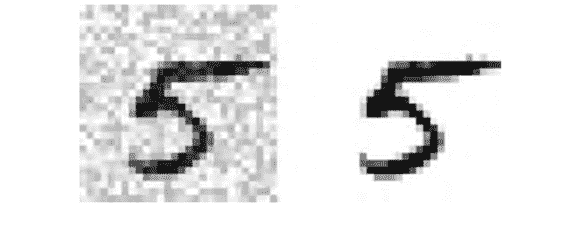knn_clf.fit(X_train_mod, y_train_mod)
clean_digit = knn_clf.predict([X_test_mod[some_index]])
plot_digit(clean_digit)## 练习

1. 尝试在 MNIST 数据集上建立一个分类器，使它在测试集上的精度超过 97%。提示：KNeighborsClassifier非常适合这个任务。你只需要找出一个好的超参数值（试一下对权重和超参数n_neighbors进行网格搜索）。
2. 写一个函数可以是 MNIST 中的图像任意方向移动（上下左右）一个像素。然后，对训练集上的每张图片，复制四个移动后的副本（每个方向一个副本），把它们加到训练集当中去。最后在扩展后的训练集上训练你最好的模型，并且在测试集上测量它的精度。你应该会观察到你的模型会有更好的表现。这种人工扩大训练集的方法叫做数据增强，或者训练集扩张。
3. 拿 Titanic 数据集去捣鼓一番。开始这个项目有一个很棒的平台：Kaggle！
4. 建立一个垃圾邮件分类器（这是一个更有挑战性的练习）：
5. 下载垃圾邮件和非垃圾邮件的样例数据。地址是 Apache SpamAssassin 的公共数据集
6. 解压这些数据集，并且熟悉它的数据格式。
7. 将数据集分成训练集和测试集
8. 写一个数据准备的流水线，将每一封邮件转换为特征向量。你的流水线应该将一封邮件转换为一个稀疏向量，对于所有可能的词，这个向量标志哪个词出现了，哪个词没有出现。举例子，如果所有邮件只包含了"Hello","How","are", "you"这四个词，那么一封邮件（内容是："Hello you Hello Hello you"）将会被转换为向量[1, 0, 0, 1](意思是："Hello"出现，"How"不出现，"are"不出现，"you"出现)，或者[3, 0, 0, 2]，如果你想数出每个单词出现的次数。
9. 你也许想给你的流水线增加超参数，控制是否剥过邮件头、将邮件转换为小写、去除标点符号、将所有 URL 替换成"URL"，将所有数字替换成"NUMBER"，或者甚至提取词干（比如，截断词尾。有现成的 Python 库可以做到这点）。
10. 然后 尝试几个不同的分类器，看看你可否建立一个很棒的垃圾邮件分类器，同时有着高召回率和高准确率。